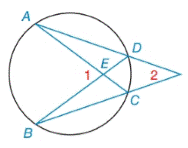Chapter 6.2, Problem 10EElementary Geometry For College St...

7th Edition
Alexander + 2 others
ISBN: 9781337614085

Solutions

Chapter
SectionElementary Geometry For College St...

7th Edition
Alexander + 2 others
ISBN: 9781337614085
Textbook Problem

Given: m ∠ 1=72 ° , m D C ⌢ = 34 ° Find: a) m A B ⌢ b) m ∠ 2To determine

(a)

To find:

mAB when m1=72° and mDC=34°.

Explanation

Theorem:

The measure of an angle formed by two chords that intersect that intersect within a circle is one-half the sum of the measure of the arcs intercepted by the angle and its vertical angle.

Calculation:

mAB when m1=72° and mDC=34°.

By Theorem,

m1=12(mAB+mDC)7

To determine

(b)

To find:

m2 when m1=72° and mDC=34°.

Still sussing out bartleby?

Check out a sample textbook solution.

See a sample solution

The Solution to Your Study Problems

Bartleby provides explanations to thousands of textbook problems written by our experts, many with advanced degrees!

Get Started

Find more solutions based on key concepts